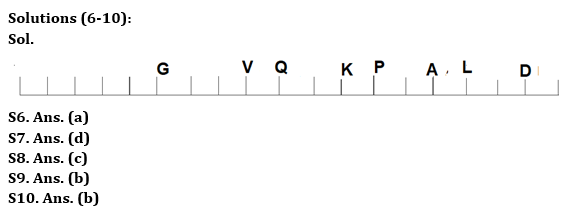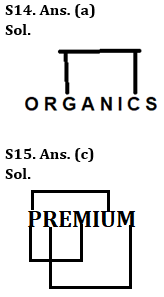Latest Banking jobs   »

# Reasoning Ability Quiz For Bank Foundation 2023 -08th May

Directions (1-5): Study the following series carefully & answer the questions:

E 7 & S 4 6 T # @ C 9 M N 1 ! D F 5 L Q \$ X 8

Q1. If all the symbols are dropped, then which element will be second to the right of eighth element from left end?
(a) 9
(b) M
(c) N
(d) 1
(e) D

Q2. How many symbols lies between 2nd number from left end and 2nd number from right end?
(a) 5
(b) 4
(c) 3
(d) 2
(e) 1

Q3. If all the numbers are dropped, then which element will be second to the left of twelfth element from left end?
(a) !
(b) 1
(c) D
(d) 5
(e) None of the above

Q4. How many such consonants are in the above arrangement, each of which is immediately preceded by a vowel or immediately followed by a symbol but not both?
(a) None
(b) One
(c) Two
(d) Three
(e) Four

Q5. How many such consonants are in the above arrangement, each of which is immediately followed by a vowel but not immediately preceded by a number?
(a) None
(b) Two
(c) Three
(d) Four
(e) Five

Directions (6-10): Answer the questions based on the information given below:
Certain number of persons sits in a straight row facing north. V doesn’t sit adjacent to L. Five persons sit between K and D. A sits exactly between K and D. Five persons sit between A and V. L sits 4th to the right of K. D sits 2nd from one of the extreme ends. Two persons sit between V and G. Number of persons to the right of G is two more than those to the left of K. P sits 3rd to the right of Q who sits exactly in the middle of the row.

Q6. Who sits adjacent to P?
(a) K
(b) A
(c) None of these
(d) L
(e) G

Q7. How many persons sit to the left of G?
(a) Six
(b) Nine
(c) Four
(d) Five
(e) None of these

Q8. How many persons sit in the row?
(a) 21
(b) 17
(c) 19
(d) 15
(e) None of these

Q9. Who among the following doesn’t sit to the left of Q?
I. V
II. K
III. G
(a) Only I
(b) Only II
(c) Only III
(d) Only I and II
(e) Only II and III

Q10. What is the position of Q with respect to K?
(a) 3rd to the left
(b) 2nd to the left
(c) 4th to the right
(d) 2nd to the right
(e) None of these

Q11. If it is possible to make a meaningful word from 2nd, 3rd, 5th and 6th letters of word “COMPREHENSIVE”, then which will be the 3rd letter of that meaningful word? If no such meaningful word is formed, then mark the answer as ‘Y’. If more than one meaningful word is formed, then mark the answer as ‘X’.
(a) Y
(b) O
(c) E
(d) R
(e) X

Q12. Which of the following letter will become the exact middle letter, if all the letters of the word “COPYRIGHT” are arranged in reverse alphabetical order from left to right?
(a) P
(b) H
(c) O
(d) R
(e) None of the above

Q13. If it is possible to make a meaningful word from 1st, 3rd, 4th and 6th letters of word “ANTIFLAME”, then which will be the 3rd letter of that meaningful word? If no such meaningful word is formed, then mark the answer as ‘Y’. If more than one meaningful word is formed, then mark the answer as ‘X’.
(a) Y
(b) T
(c) A
(d) I
(e) X

Q14. How many pairs of letters are there in the word “ORGANICS” which has as many letters between them in the word as they have in the alphabetical series (both in forward and backward direction)?
(a) One
(b) Two
(c) Three
(d) Four
(e) None of the above

Q15. How many pairs of letters are there in the word “PREMIUM” which has as many letters between them in the word as they have in the alphabetical series (both in forward and backward direction)?
(a) One
(b) Two
(c) Three
(d) Four
(e) None of the above

Solutions

Solutions (1-5):
S1. Ans. (c)
Sol. After dropping symbols: E 7 S 4 6 T C 9 M N 1 D F 5 L Q X 8.
So, N will be second to the right of eighth element from left end. Hence, option (c) is correct answer.

S2. Ans. (c)
Sol. There are three symbols between 2nd number from left end and 2nd number from right end.

S3. Ans. (a)
Sol. After dropping numbers: E & S T # @ C M N ! D F L Q \$ X
So, ! will be second to the left of twelfth element from left end. Hence, option (a) is correct answer.

S4. Ans. (c)
Sol. Given Series – E 7 & S 4 6 T # @ C 9 M N 1 ! D F 5 L Q \$ X 8
So, there are two consonants T and Q.

S5. Ans. (a)
Sol. Given Series – E 7 & S 4 6 T # @ C 9 M N 1 ! D F 5 L Q \$ X 8
So, there is no consonant.S11. Ans. (d)
Sol. 2nd, 3rd, 5th and 6th letters of the given word are O, M, R, E respectively. So, the word ‘MORE’ is formed.

S12. Ans. (c)
Sol. If the letters are arranged in reverse alphabetical order, then the word will become= “YTRPOIHGC”. So, the middle letter will be O.

S13. Ans. (d)
Sol. 1st, 3rd, 4th and 6th letters of word are A, T, I, L. The meaningful word formed is ‘TAIL’. So, the 3rd letter is I.## FAQs

### What is the selection process of the Bank Clerk?

The selection process of the Bank Clerk is Prelims & Mains.

#### Congratulations!Union Budget 2023-24: Free PDF